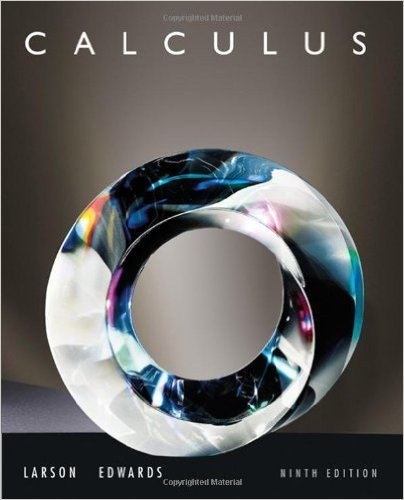×

×

# Solutions for Chapter 12: Vector-Valued Functions## Full solutions for Calculus | 9th Edition

ISBN: 9780547167022Solutions for Chapter 12: Vector-Valued Functions

Solutions for Chapter 12
4 5 0 394 Reviews
12
5
##### ISBN: 9780547167022

Calculus was written by and is associated to the ISBN: 9780547167022. This expansive textbook survival guide covers the following chapters and their solutions. Since 78 problems in chapter 12: Vector-Valued Functions have been answered, more than 157449 students have viewed full step-by-step solutions from this chapter. Chapter 12: Vector-Valued Functions includes 78 full step-by-step solutions. This textbook survival guide was created for the textbook: Calculus , edition: 9.

Key Calculus Terms and definitions covered in this textbook

P(A or B) = P(A) + P(B) - P(A and B). If A and B are mutually exclusive events, then P(A or B) = P(A) + P(B)

• Additive identity for the complex numbers

0 + 0i is the complex number zero

• Convenience sample

A sample that sacrifices randomness for convenience

• Cosecant

The function y = csc x

• Damping factor

The factor Ae-a in an equation such as y = Ae-at cos bt

• Derivative of ƒ

The function defined by ƒ'(x) = limh:0ƒ(x + h) - ƒ(x)h for all of x where the limit exists

• Equal matrices

Matrices that have the same order and equal corresponding elements.

• Explanatory variable

A variable that affects a response variable.

• Focal length of a parabola

The directed distance from the vertex to the focus.

• Heron’s formula

The area of ¢ABC with semiperimeter s is given by 2s1s - a21s - b21s - c2.

• Inverse composition rule

The composition of a one-toone function with its inverse results in the identity function.

• Inverse cotangent function

The function y = cot-1 x

• Measure of an angle

The number of degrees or radians in an angle

• Multiplication principle of probability

If A and B are independent events, then P(A and B) = P(A) # P(B). If Adepends on B, then P(A and B) = P(A|B) # P(B)

• Polar form of a complex number

See Trigonometric form of a complex number.

The distance from a point on a circle (or a sphere) to the center of the circle (or the sphere).

• Rose curve

A graph of a polar equation or r = a cos nu.

• Slope

Ratio change in y/change in x

• y-coordinate

The directed distance from the x-axis xz-plane to a point in a plane (space), or the second number in an ordered pair (triple), pp. 12, 629.